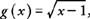## Combining and Composing Functions

You can create new functions by combining existing functions. Usually, these new functions are the result of something as simple as addition or subtraction, but functions are capable of combining in ways other than those simple binary operations.

## Arithmetic combinations

First let's look at the easiest way to create a new function from existing functions: performing basic arithmetic operations.

Example 1: If f( x) = x 2 − 2 x − 3 and g( x) = x + 1, find the following.

••Note that the domain of the new function is all real numbers except for x = −1, which is different than the domain of both f( x) and g( x).

## The composition of functions

The process of plugging one function into another is called the composition of functions. When one function is composed with another, it is usually written explicitly: f( g( x)), which is read “ f of g of x.” In other words, x is plugged into g, and that result is in turn plugged into f. Function composition can also be written using this notation: ( h · k) ( x), which is the mathematical equivalent of the statement h( k( x)).

Example 2: If f( x) = x 2 + 10 and, find the following.